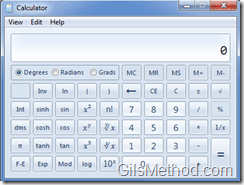# Tip: Calculate Lease Payments and Other Items with the Windows 7 Calculator

2
4886

Looking to calculate your next mortgage payment? Forget Excel, open the built-in Windows Calculator. The Calculator in Windows 7 is loaded with features. You can use one of the 4 basic calculators or use a series of worksheets where you can solve for mortgage payments or your fuel consumption, among others. The Calculator is standard with all Windows 7 installations. To locate and use the Calculator follow the instructions below.

If you have Windows 7 tips or questions, please post them below.

Note: I used Windows 7 Ultimate for this tutorial.

### Open the Calculator1. Click on the Windows Orb to open the Start Menu.

2. Type calculator into the Windows Search box.

3. Click on the Calculator icon under Programs from the search results list.

### Using the CalculatorThe plain Calculator, but wait thereâ€™s moreâ€¦1. To access the other types of calculators and the worksheets, click on View.

2. Click on the Calculator type or one of the Worksheets from the menu.You can use a Scientific Calculator.A Programmers Calculator.A Statistics Calculator.Unit Conversion â€“ Click on what you want to convert, the units from and to and you shall have a response.Want to find exactly how old you are? Enter your birth date and todayâ€™s date to find out how old you are in years, months, weeks, and days. You can also find out how many days old you are.

### Worksheets

You can use the Worksheets to calculate loan payments, lease payments, gas consumption, and their variants.Use the Mortgage Worksheet to calculate the following:

• Down Payments
• Monthly Payments
• Purchase Price
• Term (years)Use the Vehicle Lease Worksheet to calculate the following:

• Lease Period
• Lease Value
• Periodic Payments
• Residual ValuesUse the Fuel Economy (mpg) Worksheet to calculate the following:

• Distance
• Fuel Economy
• Fuel UsedUse the Fuel Economy (l/km) Worksheet to calculate the following:

• Distance
• Fuel Economy
• Fuel Used